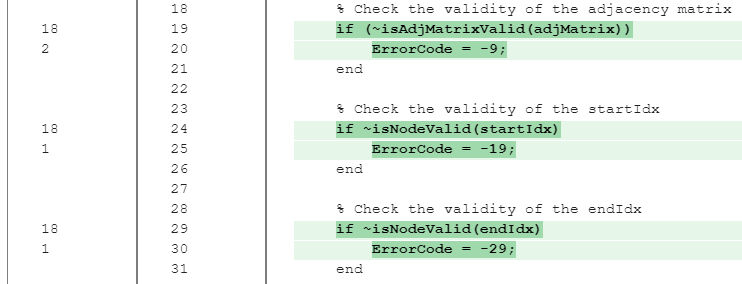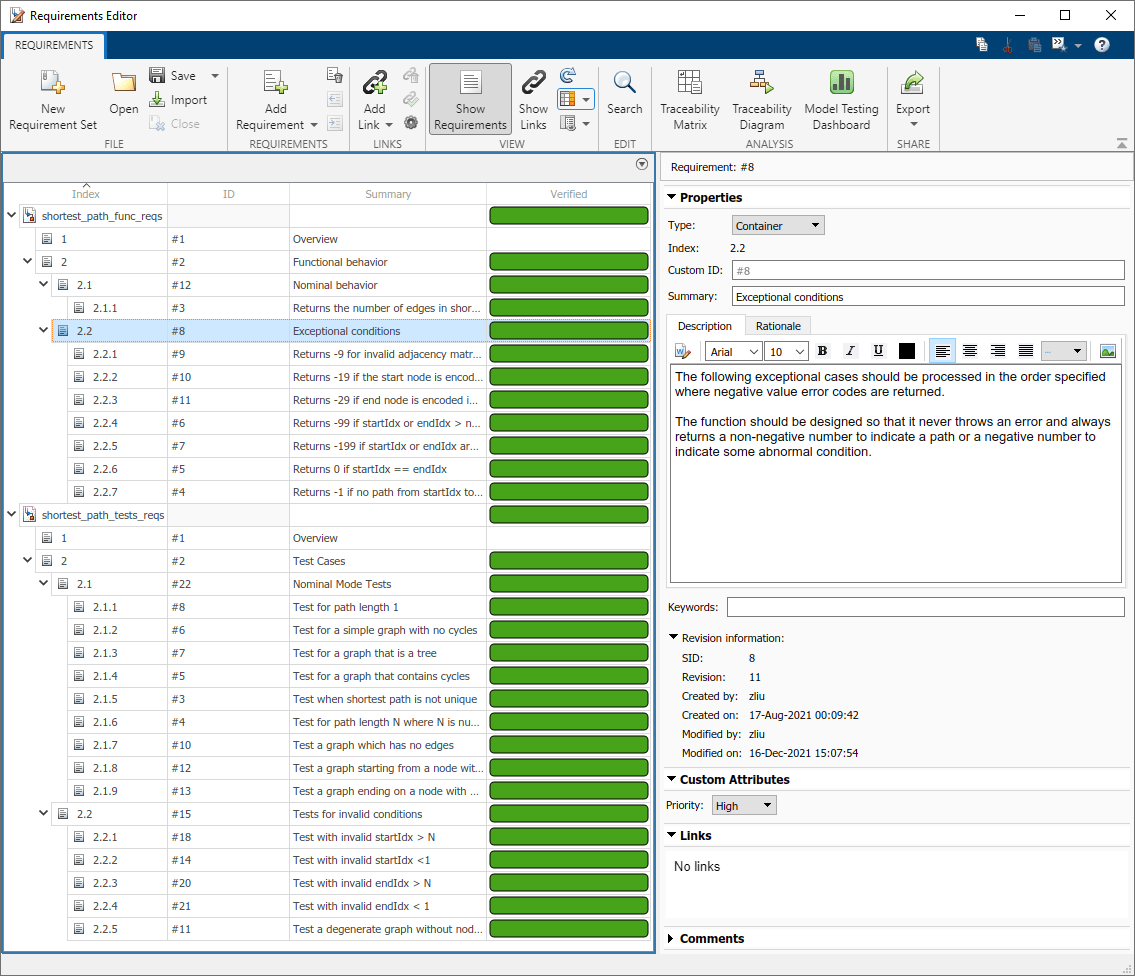# Verify a MATLAB Algorithm by Using Requirements-Based Tests

This example shows how to verify a MATLAB® algorithm by creating verification links from MATLAB code lines in functions and tests to requirements. This example uses a project that contains an algorithm to calculate the shortest path between two nodes on a graph.

Open the project.

`slreqShortestPathProjectStart`

### Examine the Project Artifacts

The project contains:

• Requirement sets for functional and test requirements, located in the `requirements` folder

• A MATLAB algorithm, located in the `src` folder

• MATLAB unit tests, located in the `tests` folder

• Links from MATLAB code lines to requirements, stored `.slmx` files located in the `src` and `tests` folders

• Scripts to automate project analysis, located in the `scripts` folder

#### Open the Functional Requirement Set

The `shortest_path_func_reqs` requirement set captures the functional behavior that the `shortest_path` function requires. The requirements describe the nominal behavior and the expected behavior for invalid conditions, such as when the inputs to the function are not valid. Open the requirement set in the Requirements Editor.

`funcReqs = slreq.open("shortest_path_func_reqs");`

#### Use the Shortest Path Function

The `shortest_path` function tests the validity of the inputs to the function and then uses the Djikstra algorithm to calculate the number of edges in the shortest path between two nodes on a graph. The inputs to the function are an adjacency matrix that represents a graph, the starting node, and the ending node. For example, consider this adjacency matrix that represents a graph with six nodes.

```A = [0 1 0 0 1 0; 1 0 1 0 0 0; 0 1 0 1 0 0; 0 0 1 0 1 1; 1 0 0 1 0 0; 0 0 0 1 0 0];```

Create a graph from the matrix and plot it.

```G = graph(A); plot(G,EdgeLabel=G.Edges.Weight)```Calculate the number of edges in the shortest path between nodes 1 and 6.

`pathLength = shortest_path(A,1,6)`
```pathLength = 3 ```

#### Open the Test Requirement Set

The `shortest_path_tests_reqs` requirement set contains test requirements that describe the functional behavior that must be tested by a test case. The test requirements are derived from the functional requirements. There are test requirements for the nominal behavior and for the invalid conditions. Open the requirement set in the Requirements Editor.

`testReqs = slreq.open("shortest_path_tests_reqs");`

The class-based MATLAB unit tests in `graph_unit_tests` implement the test cases described in `shortest_path_tests_reqs`. The class contains test methods based on the test requirements from `shortest_path_tests_reqs`. The class also contains the `verify_path_length` method, which the test cases use as a qualification method to verify that the expected and actual results are equal. The class also contains static methods that create adjacency matrices for the test cases.

#### View the Verification Status

To view the verification status, in the Requirements Editor toolstrip, in the View section, clickColumns and select Verification Status. Three of the functional requirements and one test requirement are missing verification links. The verification status is yellow for each requirement, which indicates that the linked tests have not run.Run the tests and update the verification status for the requirement sets by using the `runTests` method.

`status1 = runTests(funcReqs);`
```Running graph_unit_tests .......... ...... Done graph_unit_tests __________ ```
`status2 = runTests(testReqs);`
```Running graph_unit_tests .......... .... Done graph_unit_tests __________ ```

The verification status is green to indicate that the linked tests passed. However, some of the requirements do not have links to tests.

### Identify Traceability Gaps in the Project

The functional and test requirements are linked to code lines in the `shortest_path` and `graph_unit_tests` files, but the traceability is not complete. Use a traceability matrix to identify requirements that are not linked to tests and to create links to make the requirements fully traceable.

#### Find the Missing Links with a Traceability Matrix

Create a traceability matrix for both requirement sets with the requirements on the top and the unit tests on the left. For more information about traceability matrices, see Track Requirement Links with a Traceability Matrix

```mtxOpts = slreq.getTraceabilityMatrixOptions; mtxOpts.topArtifacts = {'shortest_path_func_reqs.slreqx','shortest_path_tests_reqs.slreqx'}; mtxOpts.leftArtifacts = {'graph_unit_tests'}; slreq.generateTraceabilityMatrix(mtxOpts)```

In the Filter Panel, in the Top section, filter the matrix to show only the functional requirements not linked to tests by clicking:

• Top > Type > Functional

In the Left section, show only the test functions in the `graph_unit_tests` file by clicking:

• Left > Type > Function

• Left > Attributes > Test

Click Highlight Missing Links in the toolstrip.The Traceability Matrix window shows the three functional requirements and one test requirement that are missing verification links.

#### Create Verification Links for Requirements

The test requirement 2.1.3, `Test for a graph that is a tree`, is not linked to a test. A tree is a graph in which any two nodes are only connected by one path.

The test case `check_invalid_start_1` tests a tree graph by using the `graph_straight_seq` static method to create the adjacency matrix. Use the` graph_straight_seq` method to view the tree graph.

```A = graph_unit_tests.graph_straight_seq; G = graph(A); plot(G,EdgeLabel=G.Edges.Weight)```Create a link from the `Test for a graph that is a tree` requirement to the `check_invalid_start_1` test case by using the traceability matrix you previously generated.

`slreq.generateTraceabilityMatrix(mtxOpts)`

Click the cell that corresponds to the requirement and the test and select Create. In the Create Link dialog box, click Create.Update the verification status in the Requirements Editor by running the tests linked to the test requirements. The `check_invalid_start_1` test verifies the `Test for a graph that is a tree` requirement.

`status3 = runTests(testReqs);`
```Running graph_unit_tests .......... .... Done graph_unit_tests __________ ```

• Requirement 2.2.1: `Returns -9 for invalid adjacency matrices`

• Requirement 2.2.2: `Returns -19 if the start node is encoded incorrectly`

• Requirement 2.2.3: `Returns -29 if end node is encoded incorrectly`

There is a traceability gap for these requirements. You cannot fill this gap by creating links to tests because there are no tests that verify these requirements.

### Fix Coverage and Traceability Gaps by Authoring Tests

The three functional requirements that do not have links to tests do have links to lines of code in the `shortest_path` function. Run the tests with coverage to determine if those lines of code in the `shortest_path` function are covered by tests.

#### Run Tests with Coverage

Use the `RunTestsWithCoverage` script to run the tests with function and statement coverage and view the coverage in a report. For more information, see Collect Statement and Function Coverage Metrics for MATLAB Source Code.

`RunTestsWithCoverage`
```Running graph_unit_tests .......... ........ Done graph_unit_tests __________ Code coverage report has been saved to: C:\Users\jdoe\MATLAB\Projects\examples\ShortestPath\coverageReport\index.html ```

Open the coverage report. The error code statements on lines 20, 25, and 30 are not covered by tests.Note that the coverage gap for these code lines and the traceability gap for requirements 2.2.1, 2.2.2, and 2.2.3 refer to the same error codes. You can close the coverage and traceability gaps simultaneously by authoring tests for these lines of code and creating links to the requirements.

#### Improve Coverage by Authoring New Tests

Create tests that improve the coverage for the tests and verify requirements 2.2.1, 2.2.2, and 2.2.2. Open the `graph_unit_tests` test file.

`open("graph_unit_tests.m");`

These functions test the three error codes. Copy and paste the code in line 4, in the test methods section of the `graph_unit_tests` file, then save the file.

```function check_invalid_nonsquare(testCase) adjMatrix = zeros(2,3); startIdx = 1; endIdx = 1; expOut = -9; verify_path_length(testCase, adjMatrix, startIdx, endIdx, expOut, ... 'Graph is not square'); end function check_invalid_entry(testCase) adjMatrix = 2*ones(4,4); startIdx = 1; endIdx = 1; expOut = -9; verify_path_length(testCase, adjMatrix, startIdx, endIdx, expOut, ... 'Adjacency matrix is not valid'); end function check_invalid_noninteger_startnode(testCase) adjMatrix = zeros(4,4); startIdx = 1.2; endIdx = 1; expOut = -19; verify_path_length(testCase, adjMatrix, startIdx, endIdx, expOut, ... 'Start node is not an integer'); end function check_invalid_noninteger_endnode(testCase) adjMatrix = zeros(4,4); startIdx = 1; endIdx = 2.2; expOut = -29; verify_path_length(testCase, adjMatrix, startIdx, endIdx, expOut, ... 'End node is not an integer'); end ```

Rerun the tests with coverage and open the coverage report.

`RunTestsWithCoverage`
```Running graph_unit_tests .......... ........ Done graph_unit_tests __________ Code coverage report has been saved to: C:\Users\jdoe\MATLAB\Projects\examples\ShortestPath\coverageReport\index.html ```

The tests now cover the error code statements.However, there is a statement on line 97 that the tests do not cover. The conditions that require the tests to cover the statement on line 97 also cause the `return` on line 87 to execute, which means that the statement on 97 is not reachable and is dead logic.#### Fix Requirement Traceability Gaps

Regenerate the traceability matrix, apply the same filters from before, then click Highlight Missing Links in the toolstrip.

`slreq.generateTraceabilityMatrix(mtxOpts)`

• Top > Type > Functional

• Left > Type > Function

• Left > Attributes > Test

Create links between the error code requirements and the new tests.Update the verification status in the Requirements Editor by re-running the tests linked to both requirement sets.

`status4 = runTests(funcReqs);`
```Running graph_unit_tests .......... ...... Done graph_unit_tests __________ ```
`status5 = runTests(testReqs);`
```Running graph_unit_tests .......... .... Done graph_unit_tests __________ ```

All requirements have links to tests and all tests pass.### Trace Requirements in Generated Code

Use Embedded Coder® to generate code from the `shortest_path` algorithm and include requirements comments that allow you to trace the requirements in the generated code. For more information, see Requirements Traceability for Code Generated from MATLAB Code.

Create a code configuration object to generate code with a LIB build type.

`cfg = coder.config("lib","ecoder",true);`

Enable the code configuration parameter to include requirements comments in the generated code.

`cfg.ReqsInCode = true;`

Use `coder.typeof` (MATLAB Coder) to define a variable-sized double array with a maximum size of 100x100 and a scalar double to use as inputs in the generated code.

```mtxType = coder.typeof(ones(100,100),[],1); scalarDblType = coder.typeof(1);```

Generate C code from the `shortest_path` algorithm with the specified code configuration parameters and input types. Create a code generation report and launch the report.

`codegen shortest_path -config cfg -args {mtxType, scalarDblType, scalarDblType} -launchreport`
```Code generation successful: View report ```The `shortest_path.c` file contains comments with the summary of the linked requirement, the full file path of the `shortest_path.m` file, and the linked code lines.